Function Repository Resource:

# RandomBins

Distribute a list into random bins

Contributed by: Ed Pegg Jr
 ResourceFunction["RandomBins"][list,n] randomly distributes the elements of list into n bins.

## Examples

### Basic Examples (1)

Randomly put the numbers 1 to 10 into three bins:

 In:=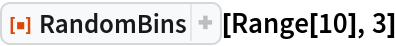Out=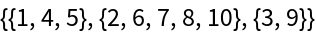### Scope (1)

Place a set of Tuples into four bins:

 In:=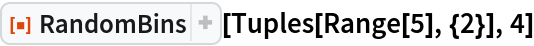Out=### Possible Issues (1)

Since the process is random, there may be fewer bins returned than the number of bins requested:

 In:=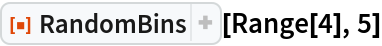Out=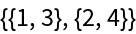### Neat Examples (1)

Randomly split the K9 complete graph into three graphs:

 In:=Out=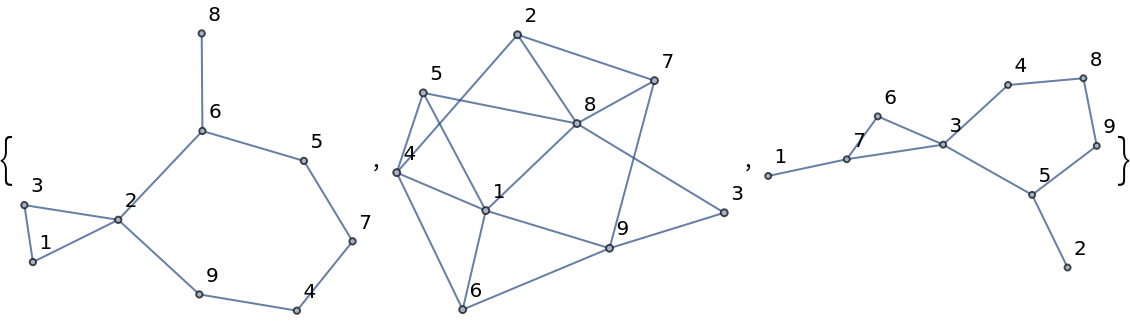## Version History

• 1.0.0 – 01 September 2021